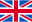# Cross-Section Values

### TB-AQS

With the TB-AQS module cross-section values can be calculated for a number of standard cross-sections..

to the shop

Discover now more programs from the section general Toolboxes!

##### Volume determination for
• square cross section
• square cross-section
• rectangular hollow profile
• double T section
• right triangle
• equilateral triangle
• circular cross-section
• circular tube cross-section
• semicircular cross-section
• hexagon cross section
• elliptical cross-section
• cross section
• octagonal cross section
• L cross section
• T cross section
• U cross section
• PDF
• Word
• Printer

Standard output

### Events06.06.23

10:00 AM - 11:00 AM CET